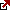# Present Value of Stock with Constant Growth

Present Value of Stock with Constant Growth

Some companies are well established and have been declaring dividends for their shareholders for many years. These companies are also showing steady growth in their share prices over many years. Hence, an investor in the company can expect similar growth and dividends for the next few decades unless there are major business conditions and company changes. Since the shareholder can expect dividends in the future, the Present Value of Stock with Constant Growth will be more than the stock’s actual listed or market value and usually includes the present value of the expected dividends.

How to calculate the present value of a stock with constant growth

The formula for calculating the present value of the stock, P0, based on dividends which are expected in the future are as follows

P0 = Div1/(r-g)

Div1 is the estimated dividend for the next period, r is the required rate of return, and g is the growth rate.

This formula assumes that the company will always grow, though some investors may question this assumption. There are also other valuing stocks for companies that have stable growth, which are making similar assumptions; hence this method is considered. Discounting the dividend is one way to value stocks using the present value of future earnings or cash flow.

Definition

In addition to the stock’s market price, the value of a stock that is growing in value consistently and offering dividends should consider the dividends which the investor will receive. The present value of these dividends is calculated and added to the stock price. The formula for calculating the growth rate g is as follows: g = Retention ratio X ROE, the return on equity is considered for the average stockholder, and retention ratio is the ratio of the company’s net income growth. Investors and others should be aware that the growth rate cannot be negative forever, though it may be negative for some time. After analysis, the right method should be selected.

Calculating the return rate required.

Some multiple options or formulae can be used to calculate the required returns rate while valuing a stock that has grown consistently. One of the more popular methods is the pricing model using capital assets.

Expected return = a + beta(rm – rf) + e

This model of calculating returns considers the overall returns for the market and the stock’s risk compared to the overall market risk rm. If the stock is riskier, the returns could be less than the market risk.

Another method used to calculate the required returns is based on calculating the present value of the companies’ expected dividends. The method assumes that the company will grow perpetually, and the formula is rearranged to calculate the returns. After rearranging, the formula is as follows r = (D/P0) + g where D is the dividend declared. This formula combines the dividend yield and growth rate.

Another theory is based on arbitrage pricing, which is similar to the capital asset model in some ways. However, this uses the different risk factors and considers each risk factor’s importance to determine the total risk for determining the stock value.

Latest posts by Fxigor (see all)

Fxigor

Igor has been a trader since 2007. Currently, Igor works for several prop trading companies. He is an expert in financial niche, long-term trading, and weekly technical levels. The primary field of Igor's research is the application of machine learning in algorithmic trading. Education: Computer Engineering and Ph.D. in machine learning. Igor regularly publishes trading-related videos on the Fxigor Youtube channel. To contact Igor write on: igor@forex.in.rs

Trade gold and silver. Visit the broker's page and start trading high liquidity spot metals - the most traded instruments in the world.Diversify your savings with a gold IRA.

Gold & silver portfolio-building assistance from product professionals.

If you want to trade stocks try: